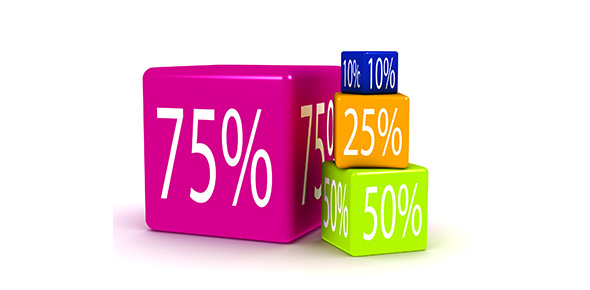Percentage Quiz With MCQ Questions

10 Questions | Total Attempts: 785SettingsBy definition, a percentage is a portion of a given total multiplied by 100. Percentages give a better measure of quantities both for analysis and presentation needs. Take up the quiz below and see how much you know on the subject.

• 1.
What is 50% of 60 ?
• 2.
Calculate  25%  of 80
• 3.
I won £300 on the lottery. I gave away 10%.  How much did I give away?
• 4.
What is 10 %  of  £80.00
• 5.
There is a 25% discount on all items today.   The total of your items is £220.    How much discount do you get?
• 6.
A beauty treatment costs £50.   If you get 25% discount, how much discount do you receive.?
• 7.
If the treatment costs £60 and you receive 25% discount, how much would you pay in total?
• 8.
The cost of  a car is £12,000.  The deposit is 20%.     How much deposit do you pay?
• 9.
If the car is £9,000 and the discount is 30%, how much discount will you get ?
• 10.
The car costs £10,000 and you get 25% discount.  How much will you pay for the car after the discount?
Related TopicsBack to top